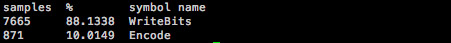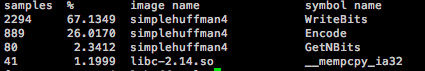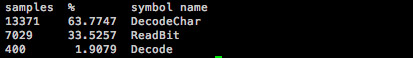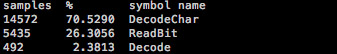# Optimizing Huffman, part 3

So far, we’ve found a number of fairly low-cost (in terms of code and complexity) ways to tune the Huffman program for better performance.

Still, after thinking about the problem for a bit, I did see some other potential areas for improvement.  In particular, the fact that the encoding process was being done essentially bit-by-bit (appending 1 bit at a time to the buffer) seemed inefficient.  If there was only some way to append a whole bunch of bits at once (i.e. the entire encoding for a given character)…

simplehuffman4.c does precisely this.

The first issue is that the encodings are stored as ASCII strings.  While convenient to work with, the data actual being written to the disk are the binary bit-representation of those strings.  Clearly, there is no direct way to overlay an ASCII string onto a buffer filled with binary bitstrings.

So I first changed the encoding table to store actual bit strings.  Rather than ASCII strings, I used integers, where the bits on in the integer corresponded exactly to those bits used in the bitstring.  If I’d used 8-bit integers, the representation for ‘11111111’ would have been 255 (all bits on).

Unlike with the ASCII strings, there is no convenient way to find the end of a bitstring directly just by looking at it.  For example, the bitstring 0110 and 011000 would have essentially the same representation (assuming one used 0 bits for the unused bits).  My bitstring format thus has to include a length, so I wound up using a pair of integers, one to hold the bits, the other to hold the length (in bits).

For convenience sake, I chose to left-align the bits, meaning that for a bitstring of length 9, I used the 9 leftmost bits in the integer, with all the other bits set to 0.

Because I use a single integer to store the bits, bitstrings are limited to a maximum length of 32 (the size of an int, in bits).  In theory, this could be a problem as encoding for 256-bit (8 byte) values could be as long as 257 bits.  However in practice none of the files I fed the program had a problem, so it seemed like a fair compromise to make.

The new process for encoding became something as follows:

1) Read in the next character from the input buffer.

2) Look up that character’s encoding in the table.

3) Overlay the bits of that character’s encoding onto our output buffer (at the correct place).

4) Repeat.

The overlaying step is the trickiest part of the process but even that is basically just a logical shift followed by a bitwise or (or in certain cases, 2).  The advantage, particularly as the bitstring gets longer, seemed like it should be quite large.

Once again, I ran the before and after code through the profiler for the case of encoding a file.

Before:After:WriteBits has dropped substantially compared to the other function calls.   At first glance it looks like Encode may have gotten slower, but in fact the number of samples suggests that Encode is taking up much more of the runtime because things are so much faster overall.  You can do the percentages, but it turns out that the new version is no less than 2.5x faster at encoding!

Did we also improve when decoding?

Before:After:Slightly.  As it happens, the ReadBit procedure did drop slightly, although the gain was partially offset by overhead in other routines.  This too makes a certain amount of sense, since all the work we did with the representation was designed to speed up the encoding end of things.

Can decoding be sped up much?  My hunch is probably not.  As long as decoding requires walking the Huffman tree, the speed of decoding is going to be significantly slower than encoding, since encoding is basically a single operation for many bits, whereas decoding requires an action for every bit.